### Solving the HO Differential Equation *

The differential equation for the 1D Harmonic Oscillator is.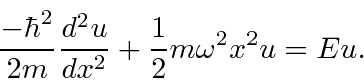By working with dimensionless variables and constants, we can see the basic equation and minimize the clutter. We use the energy in terms of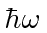.We define a dimensionless coordinate.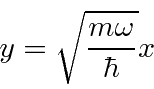The equation becomes.(Its probably easiest to just check the above equation by substituting as below.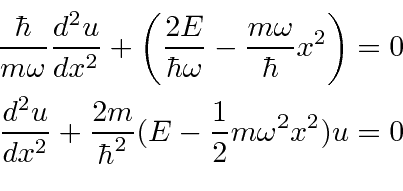It works.)

Now we want to find the solution for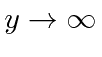.becomeswhich has the solution (in the largelimit)This exponential will dominate a polynomial asso we can write our general solution aswhere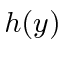is a polynomial.

Take the differential equationand pluginto it to getThis is our differential equation for the polynomial.

Writeas a sum of terms.Plug it into the differential equation.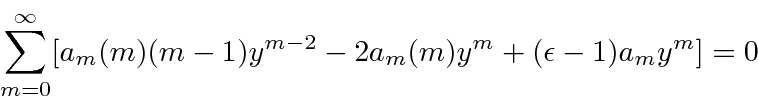We now want ot shift terms in the sum so that we see the coefficient of. To do this, we will shift the term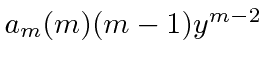down two steps in the sum. It will now show up as.(Note that in doing this shift the first term for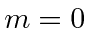and for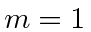get shifted out of the sum. This is OK sinceis zero foror.)

For the sum to be zero for all, each coefficient ofmust be zero.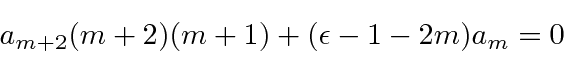Solve forand we have a recursion relation giving us our polynomial.

But, lets see what we have. For large,The series for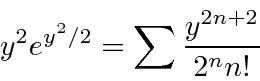has the coefficient ofequal toand the coefficient of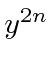equal to. If,So our polynomial solution will approachand our overall solution will not be normalizable. (Remember.) We must avoid this.

We can avoid the problem if the series terminates and does not go on to infinite.The series will terminate iffor some value of. Then the last term in the series will be of order.The acceptable solutions then satisfy the requirement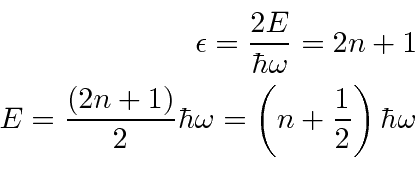Again, we get quantized energies when we satisfy the boundary conditions at infinity.

The ground state wavefunction is particularly simple, having only one term.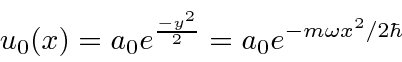Lets find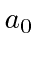by normalizing the wavefunction.Jim Branson 2013-04-22